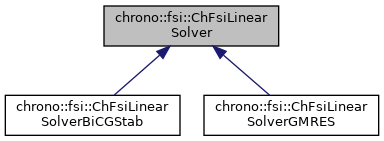chrono::fsi::ChFsiLinearSolver Class Referenceabstract

Description

Base class of the FSI GPU-based linear solvers.

This is an abstract class and specific solvers must implement the solve procedure

#include <ChFsiLinearSolver.h>

Inheritance diagram for chrono::fsi::ChFsiLinearSolver:[legend]

Public Types

enum  SolverType {
BICGSTAB = 0, GMRES, CR, CG,
SAP
}

Public Member Functions

ChFsiLinearSolver (double mrel_res=1e-8, double mabs_res=1e-4, int mmax_iter=1000, bool mverbose=false)
Constructor of the ChFsiLinearSolver. More...

virtual SolverType GetType ()=0
Return type of the solver. Derived classes should override this function.

void SetVerbose (bool mv)
Set verbose output from solver.

bool GetVerbose () const
Return whether or not verbose output is enabled.

double GetResidual ()
Return the current residual.

int GetNumIterations ()
Return the number of current Iterations.

void SetIterationLimit (int numIter)
Set and Get for the max_iter.

int GetIterationLimit ()

void SetAbsRes (double mabs_res)
Set and Get for the abs_res and.

double GetAbsRes ()

void SetRelRes (double mrel_res)

double GetRelRes ()

int GetSolverStatus ()
Return the solver status 0: unsuccessful 1: successfully converged.

virtual void Solve (int SIZE, int NNZ, double *A, unsigned int *ArowIdx, unsigned int *AcolIdx, double *x, double *b)=0
The child class should override this function and solve for x. More...

Protected Attributes

double rel_res = 1e-3

double abs_res = 1e-6

int max_iter = 500

bool verbose = false

int Iterations = 0

double residual = 1e5

int solver_status = 0

Constructor & Destructor Documentation

 chrono::fsi::ChFsiLinearSolver::ChFsiLinearSolver ( double mrel_res = 1e-8, double mabs_res = 1e-4, int mmax_iter = 1000, bool mverbose = false )

Constructor of the ChFsiLinearSolver.

Parameters
 mrel_res relative residual of the linear solver mabs_res absolute residual of the linear solver mmax_iter Maximum number of iteration of the linear solver mverbose Verbosity of solver during each solve stage

Member Function Documentation

 virtual void chrono::fsi::ChFsiLinearSolver::Solve ( int SIZE, int NNZ, double * A, unsigned int * ArowIdx, unsigned int * AcolIdx, double * x, double * b )
pure virtual

The child class should override this function and solve for x.

Parameters
 SIZE size of the matrix in Ax=b NNZ number of nonzeros in A matrix A pointer to the matrix A stored in CSR format ArowIdx accumulation of NNZ of each row of matrix A AcolIdx column index of each element of A and hence is of length NNZ x pointer to the solution vector x b pointer to the solution right hand side vector b in Ax=b

Implemented in chrono::fsi::ChFsiLinearSolverBiCGStab.